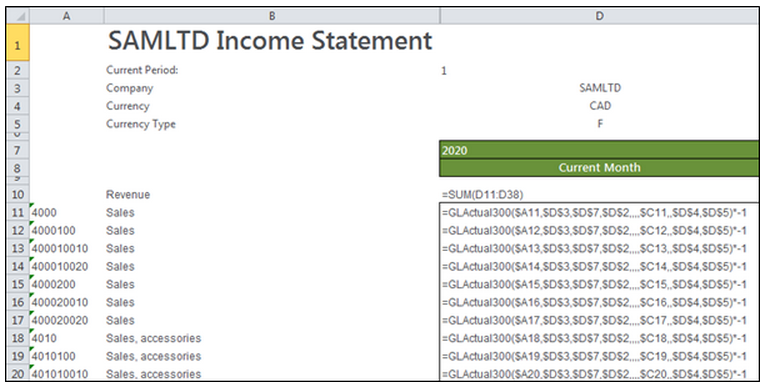# Using Formula Features in Sage Intelligence Report Designer Add-In

In this week’s tip we show you how to make use of Microsoft® Excel® formula features within the Sage 300 ERP Report Designer Add-In. Formula features that will be covered are account ranges, mathematical calculations, wildcards, cell references, and displaying cell formulas instead of values.

Using Account Ranges

A range consists of two values, where you want to retrieve data for those two values and every value between those two values.

An example would be when you want to retrieve an amount for all accounts from 4020 to 4050.  In the cell which contains the Account, you would type, 4020 TO 4050.  This will give you a summarized value for accounts 4020, 4030, 4040 and 4050.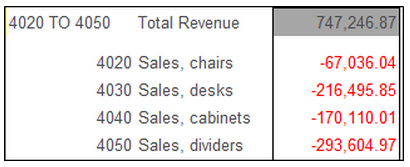TIP: Use account ranges to ensure new accounts being added to the general ledger are included in your reports.

Using Mathematical Calculations

Mathematical calculations can also be performed on all GL Accounts. This includes addition and subtraction.

For example, typing 4020 + 4050 in the cell which is linked to the Account setting will give a total figure for Account 4020 and Account 4050.

NOTE: The use of a space on either side of the + or – is required in order for the formula to be correctly recognized. Brackets are also supported, thus calculations in brackets (parenthesis) are calculated first.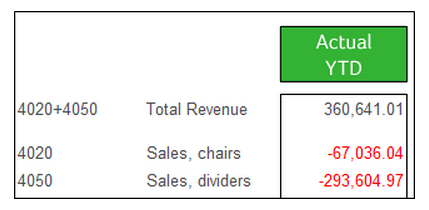Using Wildcards

Most organizations use an account structure that separates business entities into different categories. A fully qualified account contains a value for the natural segment, for example Cash or Sales, and also values for additional segments, for example Location, Division and Region.

Depending on the size of the organization, fully qualified account number segments can have different representations for different companies.

The Report Designer Add-In supports the use of special characters as a way to filter multiple account segment values, without specifically naming each one.

A question mark, (?) is a placeholder for a single character in an account segment.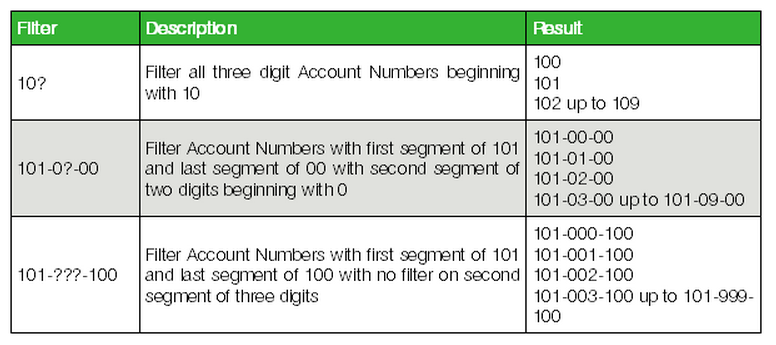An example of using wildcards in Microsoft Excel, using the GLActual300 formula, could be as follows: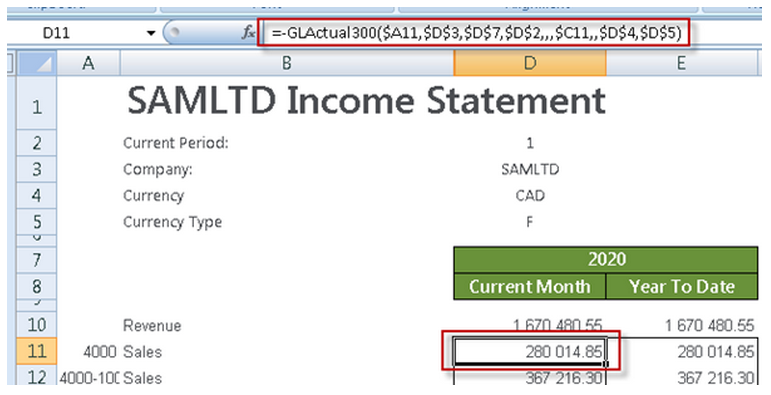Using Cell References

A cell reference identifies the location of a cell or group of cells in a worksheet. A cell reference consists of the column letter and row number that intersect at the cell’s location. When listing a cell reference, the column letter is always listed first.

The recommended method for entering data into the Sage Intelligence Reporting formulas is by using cell references.  This method makes modifying and maintaining your worksheet easier.

For example, if you wanted information for the year 2012 and you used 2011 in the Yearparameter of the GLActual300 formula, you would have to modify every formula that used the old value. If you store the year in a cell, you simply change that one cell and Microsoft Excel updates all the formulas that use that parameter.

TIP:  Excel named ranges can also be substituted for a cell reference in any formula parameter.

Using Relative or Absolute Cell References

By default, a worksheet cell reference is relative. This means that as a formula is copied and pasted to other cells, the cell references in the formula change to reflect the formula’s new location.

In contrast, an absolute cell reference does not change when its formula is copied and pasted to other cells.

An example of a relative cell reference would be A5 or B10.

An example of an absolute cell reference would be \$A\$5 or \$B\$10.

You can also mix absolute and relative cell references. An example would be copying a cell reference of \$A5, the column reference will remain, but the row reference will change to reflect the formulas new location.

If you are entering a value in your formula, be sure to include any alpha-numeric data in double-quotes (“ “). This will ensure that Microsoft Excel interprets the value as a text value and not a cell reference.

Displaying Cell Formulas instead of Values

To display all of the formulas used on your worksheet without clicking on each cell individually:

1.  Press Ctrl ~.  All of the displayed values will be replaced by the formulas used to calculate them.